We think you are located in United States. Is this correct?

# 9.2 Acid-base reactions

## 9.2 Acid-base reactions (ESCP8)

When an acid and a base react, they form a salt. If the base contains hydroxide $$(\text{OH}^{-})$$ ions, then water will also be formed. The word salt is a general term which applies to the products of all acid-base reactions. A salt is a product that is made up of the $$\color{blue}{\textbf{cation}}$$ from a $$\color{blue}{\textbf{base}}$$ and the $$\color{red}{\textbf{anion}}$$ from an $$\color{red}{\textbf{acid}}$$.

Salt

A salt is a neutral ionic compound composed of cations and anions. It is the result of an acid-base neutralisation reaction.

### Neutralisation reactions (ESCP9)

A cation is an ion (charged atom or molecule) with a positive (+) charge. An anion is an ion with a negative (-) charge.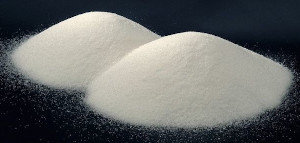Salts are not just the table salt you put on your food. A salt is any compound made up of stoichiometrically equivalent amounts of cations and anions to make a neutral, ionic compound.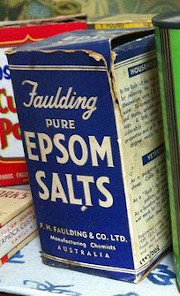Magnesium sulfate heptahydrate ($$\text{MgSO}_{4}.7\text{H}_{2}\text{O}$$), commonly known as Epsom salt, can be used as a gel to treat aches and pains, as bath salts, and has many other uses.

When an equivalent amount of acid and base react (so that neither the acid nor the base are in excess), the reaction is said to have reached the equivalence point. At this point neutralisation has been achieved.

Equivalence point

When a stoichiometrically equivalent number of moles of both reactants has been added to the reaction vessel.

To better understand stoichiometric equivalence look at the following equations:

1. $$1\color{red}{\text{HA(aq)}} + 1\color{blue}{\text{BOH(aq)}} \to$$ $$1\text{AB}(\text{aq}) + 1\text{H}_{2}\text{O}(\text{ℓ})$$

2. $$1\color{red}{\text{H}_{2}{\text{A(aq)}}} + 2\color{blue}{\text{BOH(aq)}} \to$$ $$1\text{AB}_{2}(\text{aq}) + 2\text{H}_{2}\text{O}(\text{ℓ})$$

In the first example above, a stoichiometrically equivalent number of moles is one mole of $$\color{red}{\text{HA}}$$ for every one mole of $$\color{blue}{\text{BOH}}$$. In the second example, a stoichiometrically equivalent number of moles is one mole of $$\color{red}{\text{H}_{2}{\text{A}}}$$ for every two moles of $$\color{blue}{\text{BOH}}$$.

Neutralisation

A neutralisation reaction involves an acid and a base reacting to form a salt.

Look at the following examples:

• Hydrochloric acid with sodium hydroxide

Hydrochloric acid reacts with sodium hydroxide to form sodium chloride (the salt) and water. Sodium chloride is made up of $$\text{Na}^{+}$$ cations from the base $$(\text{NaOH})$$ and $$\text{Cl}^{-}$$ anions from the acid $$(\text{HCl})$$.

$$\text{H}{\color{red}{\textbf{Cl}}}{\text{(aq)}} + \color{blue}{\textbf{Na}}{\text{OH(aq)}} \to {\color{blue}{\textbf{Na}}}{\color{red}{\textbf{Cl}}}{\text{(aq)}} + {\text{H}}_{2}{\text{O(ℓ)}}$$

• Hydrogen bromide with postassium hydroxide

Hydrogen bromide reacts with potassium hydroxide to form potassium bromide (the salt) and water. Potassium bromide is made up of $$\text{K}^{+}$$ cations from the base $$(\text{KOH})$$ and $$\text{Br}^{-}$$ anions from the acid $$(\text{HBr})$$.

$$\text{H}{\color{red}{\textbf{Br}}}{\text{(aq)}} + \color{blue}{\textbf{K}}\text{OH(aq)} \to \color{blue}{\textbf{K}}\color{red}{\textbf{Br}}{\text{(aq)}} + {\text{H}}_{2}\text{O(ℓ)}$$

• Hydrochloric acid with sodium hydrocarbonate

Hydrochloric acid reacts with sodium hydrocarbonate to form sodium chloride (the salt), water and carbon dioxide. Sodium chloride is made up of $$\text{Na}^{+}$$ cations from the base $$(\text{NaHCO}_{3})$$ and $$\text{Cl}^{-}$$ anions from the acid $$(\text{HCl})$$.

$$\text{H}{\color{red}{\textbf{Cl}}}{\text{(aq)}} + {\color{blue}{\textbf{Na}}}{\text{HCO}}_{3}{\text{(aq)}} \to \color{blue}{\textbf{Na}}\color{red}{\textbf{Cl}}{\text{(aq)}} + {\text{H}_{2}{\text{O(ℓ)}}} + {\text{CO}}_{2}{\text{(g)}}$$

You should notice that in the first two examples, the base contained $$\text{OH}^{-}$$ ions, and therefore the products were a salt and water. $$\text{NaCl}$$ (table salt) and $$\text{KBr}$$ are both salts. In the third example, $$\text{NaHCO}_{3}$$ also acts as a base, despite not having $$\text{OH}^{-}$$ ions. A salt is still formed as one of the products, but carbon dioxide ($$\text{CO}_{2}$$) is produced as well as water.

This experiment can be used for an informal assessment. It will help determine if the learners understand what happens in a neutralisation reaction. Learners are working with a strong acid and a strong base in this reaction. Concentrated, strong acids and bases can cause serious burns. Please remind the learners to be careful and wear the appropriate safety equipment when handling all chemicals, especially concentrated acids and bases. The safety equipment includes gloves, safety glasses, and protective clothing.

## Temperature changes in neutralisation reactions

### Aim

To investigate the temperature change associated with a neutralisation reaction.

### Apparatus

• $$\text{1}$$ $$\text{mol.dm^{-3}}$$ solution of sodium hydroxide ($$\text{NaOH}$$), $$\text{1}$$ $$\text{mol.dm^{-3}}$$ solution of hydrochloric acid ($$\text{HCl}$$)

• A thermometer, a beaker, a measuring cylinder

### Method

Concentrated acids and bases can cause serious burns. We suggest using gloves and safety glasses whenever you work with an acid or a base. Remember to add the acid to the water and to avoid sniffing any laboratory chemical. Handle all chemicals with care.

1. Pour $$\text{20}$$ $$\text{cm^{3}}$$ of the sodium hydroxide solution into the beaker.

2. Measure the temperature.

3. Add $$\text{5}$$ $$\text{cm^{3}}$$ of hydrochloric acid to the beaker using the measuring cylinder.

4. Repeat steps $$\text{2}$$ and $$\text{3}$$ quite quickly until the temperature no longer changes significantly.

### Observations

You should record your temperatures and the volume in a table (remember that the volume of the sodium hydroxide is constant at $$\text{20}$$ $$\text{cm^{3}}$$):

 Volume (HCl) Temperature (℃) $$\text{0}$$ room temperature

### Discussion

You should find that the reaction releases heat and so the temperature increases. After all the base has been neutralised the temperature should no longer increase. This is because the neutralisation reaction is exothermic (it releases heat). When all the base has been neutralised there is no reaction on the addition of more acid, and no more heat is released.

After the reaction has finished (the base has been neutralised) there is no more heat being produced. As a result the temperature will not rise any more. In fact, there may even be a decrease in temperature back to room temperature as the heat from the reaction vessel (the beaker) dissipates.

Salts can come in many different colours.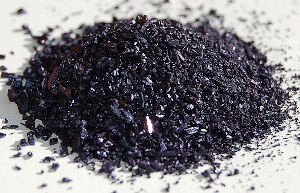$$\color{purple}{\textbf{Potassium permanganate}}$$ ($$\color{purple}{\textbf{KMnO}_{4}}$$)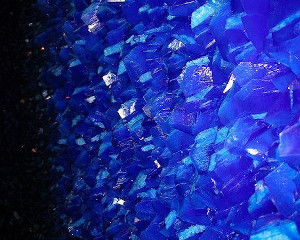$$\color{blue}{\textbf{Copper sulfate}}$$ ($$\color{blue}{\textbf{CuSO}_{4}}$$)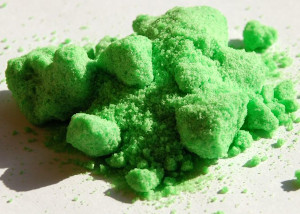$$\color{darkgreen}{\textbf{Nickel chloride}}$$ ($$\color{darkgreen}{\textbf{NiCl}_{2}}$$)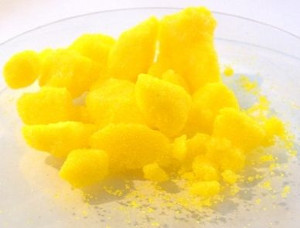$$\color{gold}{\textbf{Sodium chromate}}$$ ($$\color{gold}{\textbf{Na}_{2}{\textbf{CrO}}_{4}}$$)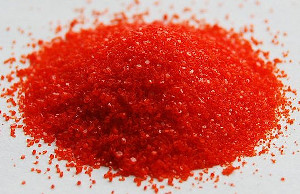$$\color{red}{\textbf{Potassim dichromate}}$$ ($$\color{red}{\textbf{K}_{2}{\textbf{Cr}}_{2}{\textbf{O}}_{7}}$$)

Neutralisation reactions are very important in every day life. Below are some examples:

• Domestic uses

Calcium oxide $$(\text{CaO})$$ is used to neutralise acidic soil. Powdered limestone $$(\text{CaCO}_{3})$$ can also be used, but its action is much slower and less effective. These substances can also be used on a larger scale in farming and in rivers.

• Biological uses

Hydrochloric acid $$(\text{HCl})$$ in the stomach plays an important role in helping to digest food. It is important to note that too much acid in the stomach may lead to the formation of ulcers in cases where the stomach lining is damaged (e.g. by an infection).

Antacids (which are bases) are taken to neutralise excess stomach acid, to prevent damage to the intestines. Examples of antacids are aluminium hydroxide, magnesium hydroxide ('milk of magnesia') and sodium bicarbonate ('bicarbonate of soda').

• Industrial uses

Alkaline calcium hydroxide (limewater) is used to absorb harmful acidic $$\text{SO}_{2}$$ gas that is released from power stations and from the burning of fossil fuels.

Please do not use a base to neutralise an acid if you spill some on yourself during an experiment. A strong base can burn you as much as a strong acid. Rather wash the area thoroughly with water.

Bee stings are acidic and have a pH between $$\text{5}$$ and $$\text{5,5}$$. They can be soothed by using substances such as calomine lotion, which is a mild alkali based on zinc oxide. Bicarbonate of soda, or soap, can also be used. The alkalis help to neutralise the acidic bee sting and relieve some of the itchiness.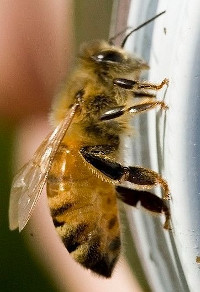## Acids and metal compounds

Research the reactions that occur when an acid is added to the following compounds:

• a metal

• a metal hydroxide

• a metal oxide

• a metal carbonate

• a metal hydrogen carbonate

Write a report which includes:

• The general equation (in words) of each reaction.

• A description of what is happening in this reaction.

• An example of this type of reaction in the form of a balanced equation.

The following is an example of how the learners acids and metals compounds report could look:

• Acid + metal $$\to$$ salt + hydrogen

A dilute acid is added to a pure metal to form a salt and hydrogen gas. The salt is formed by the metal cation and the anion from the acid. In this reaction the metal is acting as a base. For example:

$$\color{red}{\text{hydrochloric acid}} + \color{blue}{\text{zinc}} \to \color{blue}{\text{zinc}} \color{red}{\text{ chloride}}$$ + hydrogen

$$2\text{HCl}(\text{aq}) + \text{Zn}(\text{s})$$ $$\to$$ $$\text{ZnCl}_{2}(\text{aq}) + \text{H}_{2}(\text{g})$$

• Acid + metal hydroxide $$\to$$ salt + water

A dilute acid is added to a metal hydroxide to form a salt and water. The salt is formed by the metal cation and the anion from the acid. In this reaction the metal hydroxide is acting as a base. For example:

$$\color{red}{\text{hydrochloric acid}} + \color{blue}{\text{zinc hydroxide}} \to \color{blue}{\text{zinc}} \color{red}{\text{ chloride}}$$ + water

$$2\text{HCl}(\text{aq}) + \text{Zn}(\text{OH})_{2}(\text{s})$$ $$\to$$ $$\text{ZnCl}_{2}(\text{aq}) + 2\text{H}_{2}\text{O}(\text{ℓ})$$

• Acid + metal oxide $$\to$$ salt + water

A dilute acid is added to a metal oxide to form a salt and water. The salt is formed by the metal cation and the anion from the acid. In this reaction the metal oxide is acting as a base. For example:

$$\color{red}{\text{hydrochloric acid}} + \color{blue}{\text{zinc oxide}} \to \color{blue}{\text{zinc}} \color{red}{\text{ chloride}}$$ + water

$$2\text{HCl}(\text{aq}) + \text{ZnO}(\text{s})$$ $$\to$$ $$\text{ZnCl}_{2}(\text{aq}) + \text{H}_{2}\text{O}(\text{ℓ})$$

• Acid + metal carbonate $$\to$$ salt + water + carbon dioxide

A dilute acid is added to a metal carbonate to form a salt, water and carbon dioxide gas. The salt is formed by the metal cation and the anion from the acid. In this reaction the metal carbonate is acting as a base. For example:

$$\color{red}{\text{hydrochloric acid}} + \color{blue}{\text{zinc carbonate}} \to \color{blue}{\text{zinc}} \color{red}{\text{ chloride}}$$ + water + carbon dioxide

$$2\text{HCl}(\text{aq}) + \text{ZnCO}_{3}(\text{s})$$ $$\to$$ $$\text{ZnCl}_{2}(\text{aq}) + \text{H}_{2}\text{O}(\text{ℓ}) + \text{CO}_{2}(\text{g})$$

• Acid + metal hydrogen carbonate $$\to$$ salt + water + carbon dioxide

A dilute acid is added to a metal hydrogen carbonate to form a salt, water and carbon dioxide gas. The salt is formed by the metal cation and the anion from the acid. In this reaction the metal hydrogen carbonate is acting as a base. For example:

$$\color{red}{\text{hydrochloric acid}} + \color{blue}{\text{zinc bicarbonate}} \to \color{blue}{\text{zinc}} \color{red}{\text{ chloride}}$$ + water + carbon dioxide

$$2\text{HCl}(\text{aq}) + \text{Zn}(\text{HCO}_{3})_{2}(\text{s})$$ $$\to$$ $$>\text{ZnCl}_{2}(\text{aq}) + 2\text{H}_{2}\text{O}(\text{ℓ}) + 2\text{CO}_{2}(\text{g})$$

## The hazardous nature of acids and bases

Search for information about the following strong acids and bases:

• Hydrochloric acid ($$\text{HCl}$$)

• Sulfuric acid ($$\text{H}_{2}\text{SO}_{4}$$)

• Sodium hydroxide ($$\text{NaOH}$$)

• Potassium hydroxide ($$\text{KOH}$$)

Write a report which includes:

• The uses of these compounds in industry

• If applicable, the environmental waste that contains these compounds

• What the effect of a large spillage of these compounds would be

The following is an example of the sort of information learners should report on:

Hydrochloric acid

Hydrochloric acid is used industrially to clean rust from iron or steel as well as in the refining of ores. If released into the environment hydrochloric acid will lower the pH of any water it pollutes. This change in pH can seriously affect the growth of plants and damage ecosystems.

## Worked example 8: Determining equations from starting materials

Magnesium carbonate ($$\text{MgCO}_{3}$$) is dissolved in nitric acid ($$\text{HNO}_{3}$$). Give the balanced chemical equation for this reaction.

### What are the reactants?

An acid ($$\text{HNO}_{3}$$) and a metal carbonate ($$\text{MgCO}_{3}$$).

### What will the products be?

As this is the reaction of an acid and a metal carbonate the products will be a salt, water and carbon dioxide.

nitric acid + magnesium carbonate $$\to$$ salt + water + carbon dioxide

### What is the formula of the salt?

The cation will come from the metal carbonate ($$\text{Mg}^{2+}$$). The anion will come from the acid ($$\text{NO}_{3}^{-}$$). Due to the charges on the cation and anion there must be two $$\text{NO}_{3}^{-}$$ for every one $$\text{Mg}^{2+}$$.

Therefore the formula for the salt will be: $$\text{Mg}(\text{NO}_{3})_{2}$$.

### Write the equation for this reaction

$$\text{HNO}_{3}(\text{aq}) + \text{MgCO}_{3}(\text{s})$$ $$\to$$ $$\text{Mg}(\text{NO}_{3})_{2}(\text{aq}) + \text{H}_{2}\text{O}(\text{ℓ}) + \text{CO}_{2}(\text{g})$$

### Make sure that the equation is balanced

The equation is not balanced.

 Number on left Number on right H $$\text{1}$$ $$\text{2}$$ N $$\text{1}$$ $$\text{2}$$ O $$\text{6}$$ $$\text{9}$$ Mg $$\text{1}$$ $$\text{1}$$ C $$\text{1}$$ $$\text{1}$$

To balance this equation there needs to be two nitric acid molecules on the left hand side.

$$2\text{HNO}_{3}(\text{aq}) + \text{MgCO}_{3}(\text{s})$$ $$\to$$ $$\text{Mg}(\text{NO}_{3})_{2}(\text{aq}) + \text{H}_{2}\text{O}(\text{ℓ}) + \text{CO}_{2}(\text{g})$$

 Number on left Number on right H $$\text{2}$$ $$\text{2}$$ N $$\text{2}$$ $$\text{2}$$ O $$\text{9}$$ $$\text{9}$$ Mg $$\text{1}$$ $$\text{1}$$ C $$\text{1}$$ $$\text{1}$$

The equation is now balanced.

temp text

## Worked example 9: Determining equations from starting materials

Hydroiodic acid ($$\text{HI}$$) is added to solid potassium hydroxide ($$\text{KOH}$$). Give the balanced chemical equation for this reaction.

### What are the reactants?

An acid ($$\text{HI}$$) and a base ($$\text{KOH}$$).

### What will the products be?

As this is the reaction of an acid and base (which contains a hydroxide anion) the products will be a salt and water.

hydroiodic acid + potassium hydroxide $$\to$$ salt + water

### What is the formula of the salt?

The cation will come from the base ($$\text{K}^{+}$$). The anion will come from the acid ($$\text{I}^{-}$$). Due to the charges on the cation and anion there must be one $$\text{K}^{+}$$ for every one $$\text{I}^{-}$$.

Therefore the formula for the salt will be: $$\text{KI}$$.

### Write the equation for this reaction

$$\text{HI}(\text{aq}) + \text{KOH}(\text{s})$$ $$\to$$ $$\text{KI}(\text{aq}) + \text{H}_{2}\text{O}(\text{ℓ})$$

This can also be written:

$$\text{HI}(\text{aq}) + \text{KOH}(\text{s})$$ $$\to$$ $$\text{K}^{+}(\text{aq}) + \text{I}^{-}(\text{aq}) + \text{H}_{2}\text{O}(\text{ℓ})$$

### Make sure that the equation is balanced

The equation is balanced.

## Worked example 10: Determining equations from starting materials

Sulfuric acid ($$\text{H}_{2}\text{SO}_{4}$$) and ammonia ($$\text{NH}_{3}$$) are combined. Give the balanced chemical equation for this reaction.

### What are the reactants?

An acid ($$\text{H}_{2}\text{SO}_{4}$$) and a base ($$\text{NH}_{3}$$).

### What will the products be?

As this is the reaction of an acid and a base (with no hydroxide anion), there will be a salt as a product. There may or may not be another product.

sulfuric acid + ammonia $$\to$$ salt (+ maybe another product)

### What is the formula of the salt?

The cation will come from the base ($$\text{NH}_{4}^{+}$$). The anion will come from the acid ($$\text{SO}_{4}^{2-}$$). Due to the charges on the cation and anion there must be two $$\text{NH}_{4}^{+}$$ for every one $$\text{SO}_{4}^{2-}$$.

Therefore the formula for the salt will be: $$(\text{NH}_{4})_{2}\text{SO}_{4}$$.

### Write the equation for this reaction so far

$$\text{H}_{2}\text{SO}_{4}(\text{aq}) + \text{NH}_{3}(\text{g})$$ $$\to$$ $$(\text{NH}_{4})_{2}\text{SO}_{4}(\text{aq})$$ (+ maybe another product)

### Determine if there will be another product

There are no atom types that are not accounted for on both sides of the equation, therefore it is unlikely that there will be another product. If the equation can be balanced then there is no other product.

### Make sure that the equation is balanced

The equation is not balanced.

 Number on left Number on right H $$\text{5}$$ $$\text{8}$$ S $$\text{1}$$ $$\text{1}$$ O $$\text{4}$$ $$\text{4}$$ N $$\text{1}$$ $$\text{2}$$

To balance this equation there needs to be two ammonia molecules on the left hand side.

$$\text{H}_{2}\text{SO}_{4}(\text{aq}) + 2\text{NH}_{3}(\text{g})$$ $$\to$$ $$(\text{NH}_{4})_{2}\text{SO}_{4}(\text{aq})$$

 Number on left Number on right H $$\text{8}$$ $$\text{8}$$ S $$\text{1}$$ $$\text{1}$$ O $$\text{4}$$ $$\text{4}$$ N $$\text{2}$$ $$\text{2}$$

The equation is now balanced.

## Reactions of acids and bases

Textbook Exercise 9.4

Write balanced equations for these acid and metal reactions:

Hydrochloric acid and calcium

acid ($$\text{HCl}$$) + metal ($$\text{Ca}$$) $$\to$$ salt + hydrogen

Anion (from acid) is $$\text{Cl}^{-}$$, cation (from metal) is $$\text{Ca}^{2+}$$.

Therefore salt is $$\text{CaCl}_{2}$$.

$$\text{HCl}(\text{aq}) + \text{Ca}(\text{s})$$ $$\to$$ $$\text{CaCl}_{2}(\text{aq}) + \text{H}_{2}(\text{g})$$

To balance this equation there must be two $$\text{HCl}$$ molecules.

$$2\text{HCl}(\text{aq}) + \text{Ca}(\text{s})$$ $$\to$$ $$\text{CaCl}_{2}(\text{aq}) + \text{H}_{2}(\text{g})$$

Nitric acid and magnesium

acid ($$\text{HNO}_{3}$$) + metal ($$\text{Mg}$$) $$\to$$ salt + hydrogen

Anion (from acid) is $$\text{NO}_{3}^{-}$$, cation (from metal) is $$\text{Mg}^{2+}$$.

Therefore salt is $$\text{Mg}(\text{NO}_{3})_{2}$$.

$$\text{HNO}_{3}(\text{aq}) + \text{Mg}(\text{s})$$ $$\to$$ $$\text{Mg}(\text{NO}_{3})_{2}(\text{aq}) + \text{H}_{2}(\text{g})$$

To balance this equation there must be two $$\text{HNO}_{3}$$ molecules.

$$2\text{HNO}_{3}(\text{aq}) + \text{Mg}(\text{s})$$ $$\to$$ $$\text{Mg}(\text{NO}_{3})_{2}(\text{aq}) + \text{H}_{2}(\text{g})$$

Write balanced equations for these acid and metal hydroxide reactions:

Hydrochloric acid and magnesium hydroxide

acid ($$\text{HCl}$$) + metal hydroxide ($$\text{Mg}(\text{OH})_{2}$$) $$\to$$ salt + water

Anion (from acid) is $$\text{Cl}^{-}$$, cation (from metal) is $$\text{Mg}^{2+}$$.

Therefore salt is $$\text{MgCl}_{2}$$.

$$\text{HCl}(\text{aq}) + \text{Mg}(\text{OH})_{2}(\text{s})$$ $$\to$$ $$\text{MgCl}_{2}(\text{aq}) + \text{H}_{2}\text{O}(\text{ℓ})$$

To balance this equation there must be two $$\text{HCl}$$ molecules and two $$\text{H}_{2}\text{O}$$ molecules.

$$2\text{HCl}(\text{aq}) + \text{Mg}(\text{OH})_{2}(\text{s})$$ $$\to$$ $$\text{MgCl}_{2}(\text{aq}) + 2\text{H}_{2}\text{O}(\text{ℓ})$$

Nitric acid and aluminium hydroxide

acid ($$\text{HNO}_{3}$$) + metal hydroxide ($$\text{Al}(\text{OH})_{3}$$) $$\to$$ salt + water

Anion (from acid) is $$\text{NO}_{3}^{-}$$, cation (from metal) is $$\text{Al}^{3+}$$.

Therefore salt is $$\text{Al}(\text{NO}_{3})_{3}$$.

$$\text{HNO}_{3}(\text{aq}) + \text{Al}(\text{OH})_{3}(\text{s})$$ $$\to$$ $$\text{Al}(\text{NO}_{3})_{2}(\text{aq}) + \text{H}_{2}\text{O}(\text{ℓ})$$

To balance this equation there must be three $$\text{HNO}_{3}$$ molecules and three $$\text{H}_{2}\text{O}$$ molecules.

$$3\text{HNO}_{3}(\text{aq}) + \text{Al}(\text{OH})_{3}(\text{s})$$ $$\to$$ $$\text{Al}(\text{NO}_{3})_{3}(\text{aq}) + 3\text{H}_{2}\text{O}(\text{ℓ})$$

Write balanced equations for these acid and metal oxide reactions:

Hydrochloric acid and aluminium oxide

acid ($$\text{HCl}$$) + metal oxide ($$\text{Al}_{2}\text{O}_{3}$$) $$\to$$ salt + water

Anion (from acid) is $$\text{Cl}^{-}$$, cation (from metal) is $$\text{Al}^{3+}$$.

Therefore salt is $$\text{AlCl}_{3}$$.

$$\text{HCl}(\text{aq}) + \text{Al}_{2}\text{O}_{3}(\text{s})$$ $$\to$$ $$\text{AlCl}_{3}(\text{aq}) + \text{H}_{2}\text{O}(\text{ℓ})$$

To balance this equation there must be six $$\text{HCl}$$ molecules, two $$\text{AlCl}_{3}$$ molecules, and three $$\text{H}_{2}\text{O}$$ molecules.

$$6\text{HCl}(\text{aq}) + \text{Al}_{2}\text{O}_{3}(\text{s})$$ $$\to$$ $$2\text{AlCl}_{3}(\text{aq}) + 3\text{H}_{2}\text{O}(\text{ℓ})$$

Sulfuric acid and magnesium oxide

acid ($$\text{H}_{2}\text{SO}_{4}$$) + metal oxide ($$\text{MgO}$$) $$\to$$ salt + water

Anion (from acid) is $$\text{SO}_{4}^{2-}$$, cation (from metal) is $$\text{Mg}^{2+}$$.

Therefore salt is $$\text{MgSO}_{4}$$.

$$\text{H}_{2}\text{SO}_{4}(\text{aq}) + \text{MgO}(\text{s})$$ $$\to$$ $$\text{MgSO}_{4}(\text{aq}) + \text{H}_{2}\text{O}(\text{ℓ})$$

This equation is balanced.